# Selina Concise Mathematics Class 6 ICSE Solutions Chapter 30 Revision Exercise Symmetry

## Selina Concise Mathematics Class 6 ICSE Solutions Chapter 30 Revision Exercise Symmetry (Including Constructions on Symmetry)

Selina Publishers Concise Mathematics Class 6 ICSE Solutions Chapter 30 Revision Exercise Symmetry (Including Constructions on Symmetry)

### Revision Exercise Symmetry Exercise 30 – Selina Concise Mathematics Class 6 ICSE Solutions

Question 1.
State, whether true or false :Solution:Question 2.
Construct a triangle ABC, in which AB = AC = 5 cm and BC 6 cm. Draw all its lines of Symmetry.
Solution:Question 3.
Examine each of the following figures carefully, draw line(s) of symmetry in which ever figure possible :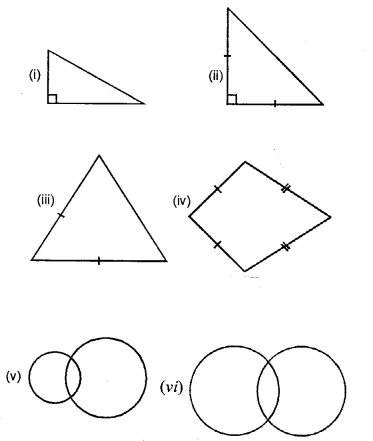Solution: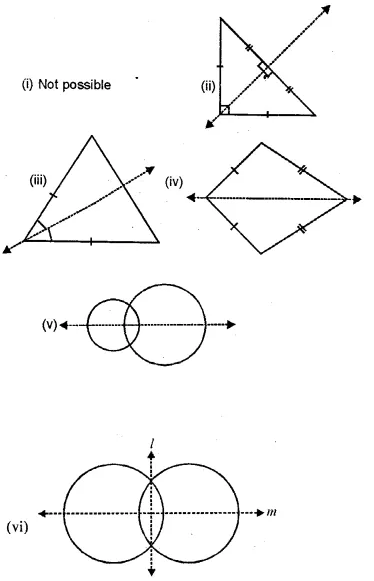Question 4.
Construct a triangle XYZ, in which XY = YZ = ZX = 4.5 cm. Draw all its lines of symmetry.
Solution:Question 5.
Construct a triangle ABC, in which AB = BC = 4 cm and ∠ABC = 60°. Draw all its lines of symmetry.
Solution: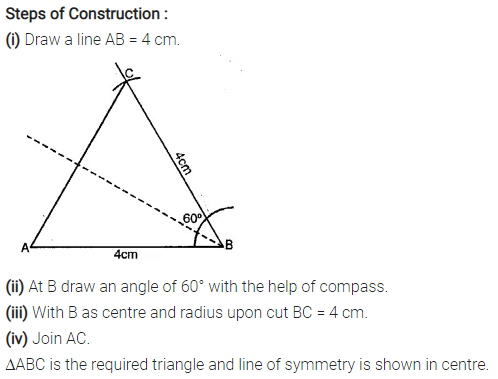Question 6.
Draw the line(s) of symmetry for each figure drawn below :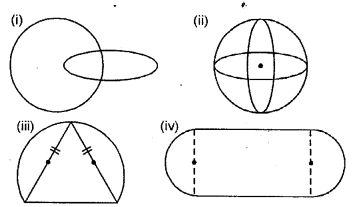Solution: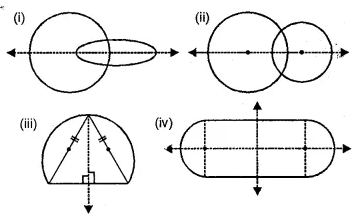Question 7.
In each of the following case, construct a point that is symmetric to the given point P with respect to the given line AB.Solution: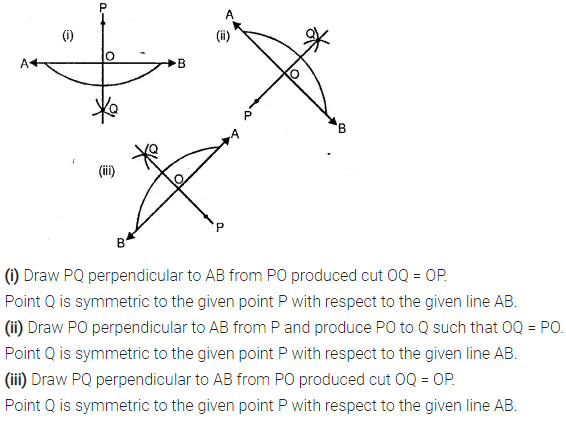Question 8.
Mark two points A and B 5.5 cm apart. Draw a line PQ so that A and B are symmetric with respect to the line PQ. Give a special name to line PQ.
Solution: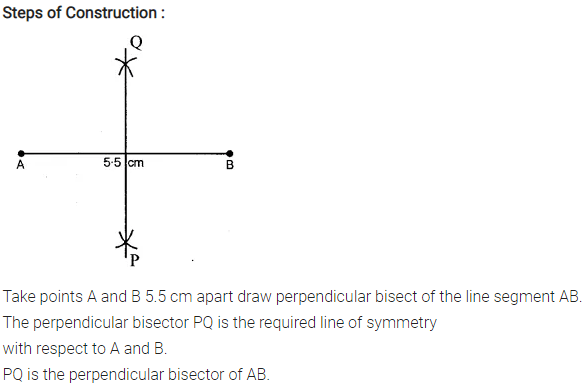Question 9.
For each letter of the English alphabet, draw the maximum possible number of lines of symmetry.
Solution: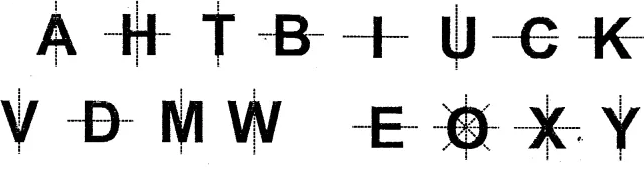Question 10.
Draw all the possible lines of symmetry for each figure given below :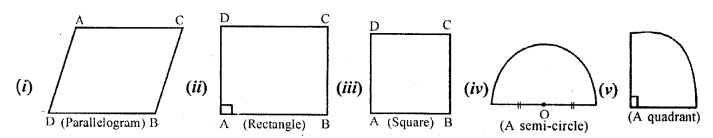Solution: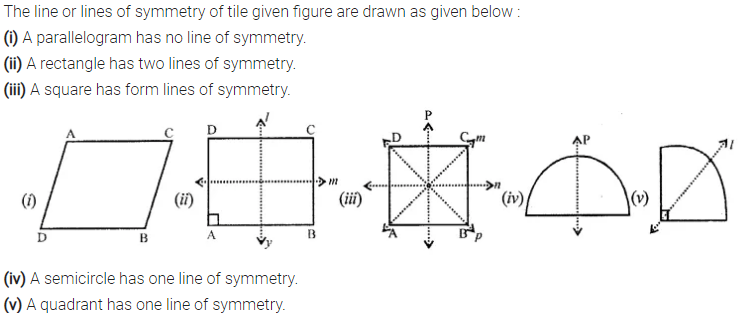Question 11.
For each shaded portion given below, draw all the possible lines of symmetry :
Solution: